PARAFAC tutorial (PortSurvey, Murphy et al. (2013))

This is a tutorial was originally published as supplementary material in Murphy et al. (2013). This tutorial only describes section 4 in Appendix A of Murphy et al. (2013). This tutorial uses a dataset consisting of 224 EEMs belonging to 26 different sites and four different cruises.

You can copy & paste the code sections in this tutorial directly into your own MATLAB script. If this document is open in the MATLAB help browser (called with `doc`), you can right-click on code sections and evaluate them line-by-line (also by pressing `F9` on windows)

If you want the code without any comments given in this tutorial, type:

``````open(which('drEEM_parafac_tutorial_portSurvey.m'))
``````

The drEEM toolbox must be installed for this command to work.

The sections of this tutorial

## 1. Getting started

Are you new to PARAFAC? Never used drEEM? Please make sure to read the documentation of the drEEM toolbox carefully. It contains links to videos that explain the theory behind PARAFAC, provides links to relevant publications, videos, and also provides detailed help for the components of drEEM. To access it, type

``````doc dreem
help dreem
``````

If you are unsure how to use a specific function, please access its documentation as follows:

``````doc functionname
% Example
doc eemreview
``````

You can also access the function documentation by typing:

``````help functionname
% Example
help eemreview
``````

Just make sure to click on the link provided in the console output.

Before we start this tutorial, we need to make sure that the drEEM toolbox is properly installed and the functions are ready to import a example dataset. This set of data is called `PortSurveyData_corrected.mat` and is stored in the drEEM toolbox folders.

If you downloaded the drEEM toolbox to your matlab user folder (the folder returned when you call `userpath`), simply call

``````cd userpath
``````

otherwise, say:

``````cd 'C:\Users\expertuser\somefolder\drEEM\' % Change this!
``````

then, we ensure proper installation of drEEM and availability of the demo files:

``````dreeminstall
``````

## 2. Data Import

To begin the tutorial, load the corrected dataset:

``````load(which('PortSurveyData_corrected.mat'))
``````

This imports ten variables into your MATLAB workspace. As a first step, we will assemble the variables we need into a single data structure using `assembledataset`:

``````mydata= assembledataset(XcQS,Ex,Em_in,'QSE',...
'site',sites,...
'rep',replicates,...
'longID',filelist_eem,...
'ID',sampleID,...
'cruise',cruises,...
'date',dates,[]);
``````

Note how theresulting dataset structure includes metadata (information describing the samples in X) invariables named site, rep ID, longID, cruise and date:

``````>> disp(mydata)
Ex: [46×1 double]
Em: [99×1 double]
X: [224×99×46 double]
IntensityUnit: 'QSE'
nEx: 46
nEm: 99
nSample: 224
site: {224×1 cell}
rep: {224×1 cell}
longID: {224×1 cell}
ID: {224×1 cell}
cruise: {224×1 cell}
date: {224×1 cell}
``````

Use classinfo to get summary information about the contents of each metadata field.

``````classinfo(mydata)
``````

We can visualise the raw data using `eemview`. This function allows us to view contour plots of raw or modelled EEMs in a customised layout and scroll forward or backward through them. View the help for `eemview` in MATLAB to learn how to use it. It is possible to control the number of plots shown per page (e.g. [3 2] = 6plots per page in 3 rows and 2 columns) and whether to number plots or label them according to the content of a metadata field(e.g. ‘site’):

``````eemview(mydata,'X',,[],[],'site') <a href="//dreem.openfluor.org/assets/ptut1_i1.png" data-lightbox="Example EEMs" data-title="Example EEMs">   <img src="//dreem.openfluor.org/assets/ptut1_i1.png" title="Example EEMs"> </a>
``````

## 3. Preprocessing

Appropriate preprocessing is essential to obtaining reliable models. There are two main goals with preprocessing:

1. correct any systematic biases in the dataset,and
2. remove data likely to harm the model while retaining as muchusefuldata as possible.

Unfortunately, the best way to preprocess a dataset is not usually obvious from the outset, such that it is often necessary to iterate the preprocessing and modelling steps in order to arrive at a stable and satisfactory PARAFAC solution. This process is illustrated in Figure 2 of the tutorial paper.When preprocessing a dataset with drEEM, it is recommended that preprocessing steps are implemented in the following order:

1. Resize the dataset to exclude noisy or contaminated Ex or Em wavelengths (if necessary).
2. Remove scatter peaksand parts of EEMs(e.g. using zap)
3. Remove outlier samples
4. Normalise the dataset

Although steps (1) and (2) can be switched in order, if smoothing is implemented before noisy data are removed, then the smoothing procedure could incorporate noisy data and be less successful as a result. Outlier data and samples should always be removed as the very last step or immediately prior to (optionally) normalising the dataset. This is for three reasons:(1) changing the Ex or Em wavelength range can change which samples are outliers, (2) if you remove any data from a normalised dataset you will not be able to reverse the normalisation accurately, (3) to obtain accurate model scores for excluded samples during the Model Export phase, a full dataset must be available that was preprocessed in exactly the same way as the modelled dataset.

The plots just displayed using eemview show non-trilinear variation (appearing as diagonal peaks) due to primary and secondary Raman and Rayleigh-Tyndall scatter. Before dealing with this, use `subdataset` to get rid of parts of the EEM that have more scatter than signal (Em>600) or that are noisy and/or likely to exert disproportionate leverage on the model (Ex<250).

``````SubData=subdataset(mydata,[],mydata.Em>600,mydata.Ex<250);
``````

`SubData` has the same contentas `mydata`, just with the chosen wavelengths removed. It also has a new field named `SubData.i`, which contains the original index of each sample. If we remove any samples, this field will track which samples from the original dataset still remain. Next, we will remove the scatter regions using `smootheem`.This function excises the scatter peaks then (optionally) interpolates across them. A number of side-by-side plots are produced by the function to make it possible to tune the input parameters so as to remove only the scatter-affected EEM regions. View the help for this function to learn how to use it. Notice you have various options about how long (if at all) to display plots.Also, note that the function must be allowed to run to completion (i.e. all plots must be viewed if the plot-viewing option is switched on), otherwise Xs will not be created. To pause between plotting each sample:

``````Xs = smootheem(SubData,[12 11],[9 16],[16 14],[20 14],[0 0 0 0],[],3382,'pause');
``````

Or to smooth the entire dataset without plotting:

``````Xs = smootheem(SubData,[12 11],[9 16],[16 14],[20 14],[0 0 0 0],[],3382,0);
``````

Please note that this step is very important for a good modeling outcome. We have therefore a dedicated smoothing tutorial available on this website.

Once satisfied with the scatter removal, use `eemview` again to look for obvious outliers.

``````eemview(Xs,'X',[3 3],[],[],'site')
``````

From plots of `Xs`, it is clear that there are two sites that represent extreme outliers relative to the others: `site = ''` (no data) and `site = '0A'`. These samples represent field blanks and tributary measurements, respectively, whereas the rest of the samples came from the Bay proper. It was fine that we did not remove them earlier, because having samples with low signals can be useful when tuning the parameters used to locate scatter peaks. However, they are outliers to the Bay dataset which is likely to present problems for PARAFAC, so it is appropriate to remove them now. We can remove them by name using `subdataset`.

``````Xin=subdataset(Xs,{'site','','0A'},[],[]);
``````

As an alternative to removing high-leverage samples (which could cause a significant loss of useful information, especially for a small dataset), it is possible to remove parts of samples containing faulty data using `zap`.

For example, sample 192 features a sharp peak in emission scans collected at excitation wavelengths of 345 and 350 nm only, which is characteristics of a fluorometer error.This is especially apparent in comparison to other EEMs (samples 191 and 193) which were collected at the same time and location.

``````eemview(Xin,'X',[1 3],176,[],[],[],[],[],20)
``````

Remove just the faulty emission scans using zap.

``````Xin=zap(Xin,176,[],[345 350]);
``````

Confirm this took care of the problem.

``````eemview(Xin,'X',[1 3],176,[],[],[],[],[],20)
``````

Notice that Xin now contains a field called `Xin.Zap` containing the input parameters for this last procedure.This information (together with data tracking other procedures implemented during model preprocessing, development and validation) will be carried forward and ultimately exported to Excel along with the validated model spectra.

## 4. Exploratory data analysis

The goal of exploratory data analysis is to get a feel for a dataset without investing a great deal of time and effort in obtaining the best possible solutions. This is the time to investigate the effects of different data preprocessing, by including or excluding samples and/or wavelengths, or varying the options for dealing with scatter peaks. To start, use `outliertest` to generate preliminary models with 3 to 6 factors and identify samples that have an unusually high impact on the models. To speed up the modelling, we will use a low convergence criterion (1e-2 instead of the more common 1e-6) and only model every other excitation and emission wavelength.

``````Test1=outliertest(Xin,[2,2],3:7);
``````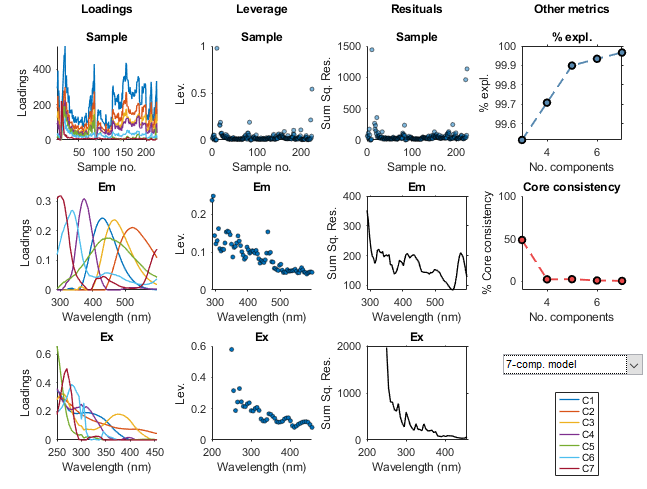Since v0.6.0, `outliertest` has been simplified. By default, nonnegativity constraints are applied, but you can easily get a feel for models that do not have this constraint enabled. For that, type:

``````Test1u=randinitanal(Xin,3:7,'constraints','unconstrained','convgcrit',1e-4,'starts',2);
``````

To view the models in Test1u with the `outliertest` plot summary, type the following and type ‘y’ when asked if plots should be produced:

``````outliertest(Test1u,[2,2],3:7)
``````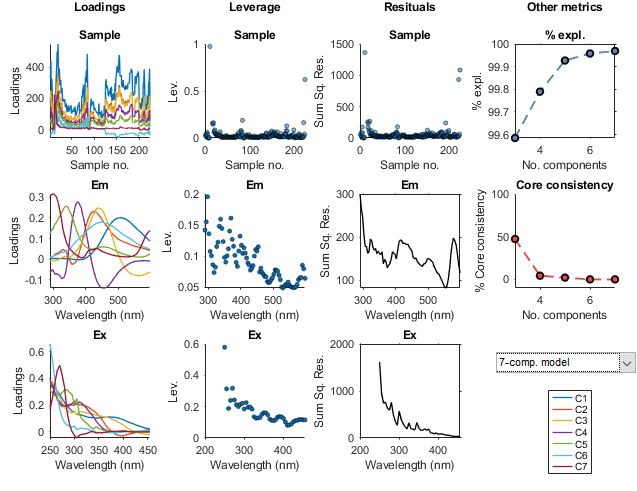View the PARAFAC components to check that they look approximately as expected. The non-negativity constraint is often necessary to obtain reasonable spectra.

Now look at correlations betweenthe components in the various nonnegative models.

``````compcorrplot(Test1,4)
compcorrplot(Test1,5)
compcorrplot(Test1,6)
``````

or

``````compcorrplot(Test1,6,[],'site')
``````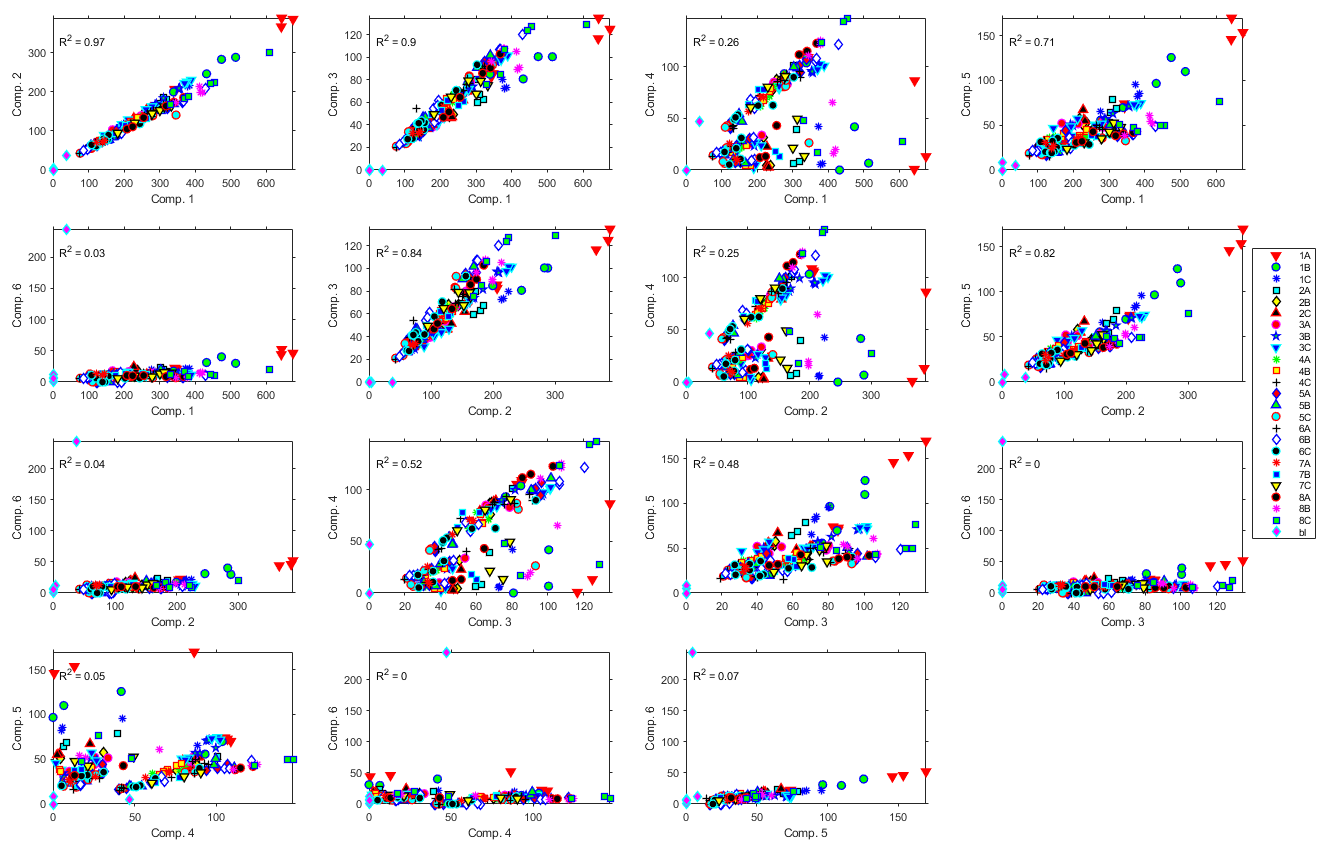In any model with more than four components, the scores (related to concentrations) of some components are very strongly correlated, suggesting that dilution is a dominant mechanism in the dataset. As is discussed in the literature, when the scores of two PARAFAC components are very highly correlated, it is very difficult for PARAFAC to accurately resolve their spectra. We will assist PARAFAC by normalising the dataset, which should greatly reduce the concentration-related colinearity and give low-concentration samples a chance to enter the model. This will help PARAFAC obtain the best possible estimates of each component’s excitation and emission spectra. Once we have determined what the spectra should be in the final model, we will project the non-normalised data on the final (normalised) model and obtain the true (non-normalised) model scores. First, develop a new dataset in which EEM intensities are normalised to unit norm. This will be the dataset modelled using PARAFAC. As the final step before exporting a validated model,we will reverse the normalisation in order to obtain the true scores.

``````Xpre=normeem(Xin);
``````

Since v0.5.0, `normeem` will ask, whether samples with very low intensities should be excluded from the dataset: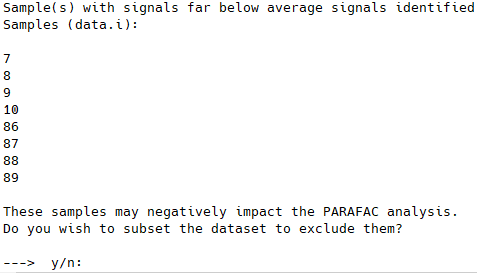Whether this makes sense is up to the user, however including such samples often results in bad PARAFAC models that focus too much on measurement noise. Here, we chose not to exclude the samples ourselves.

Perform a new outlier test on the normalised data, and view the resulting PARAFAC components:

Test1p=outliertest(Xpre,[2,2],4:7);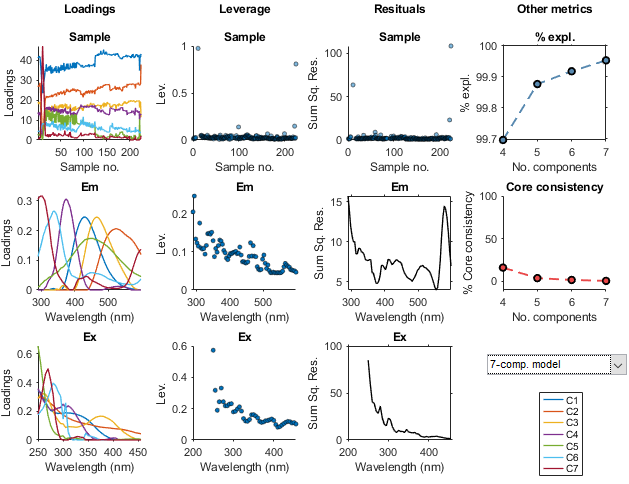``````compcorrplot(Test1p,6)
``````

It is apparent that some of the autocorrelation has been removed thanks to the normalization step.

You can always produce the outliertest plots again, even after closing the figure; here by typing:

``````outliertest(Test1p,[2,2],4:7);
``````

Some samples have high leverages and are clear outliers. We identify them by their identity using the field `i` and use logical indexing to mark them for deletion:

``````out=ismember(Xin.i,[7 8 9 10 11 86 87 88 89 215 224]);
``````

High leverage samples are not always problematic –in practice, each should be assessed individually to determine the effect of removing them.

VERY IMPORTANT: If after normalising the dataset you decide to remove samples and/or parts of samples, return to that section and repeat the steps taken to generate `Xpre`, after removing the outliers using the order of preprocessing steps described. In other words, make sure that normalising is the last thing you do to your dataset before you apply PARAFAC.

NOTE: Since PARAFAC models with low convergence criteria can differ between fits, your leverage plots may look slightly different.

``````Xin=subdataset(Xin,out,[],[]);
Xpre=normeem(Xin);
Test2=outliertest(Xpre,[2,2],5:7);
``````

There are still some high leverage samples but none appear to be severe. Leave them in for the moment, or try to improve them using zap. To view the error residuals:

``````eemview({Test2, Test2},{'X','Model6','error_residuals'},[1 3],49)
``````

For example, look at the residuals for sample 7 (`Xin.i = 17`), indicating a problem around Ex=325 nm, and in a large number of samples, a sharp peak is seen at Ex = 280-295 nm and Em= 560-576 nm. This last peak in particular could disturb the modelling because it appears in numerous samples.To view the residuals for the 5 and 6 component models in adjacent plots:

``````compare2models(Test2,5,6,0.05)
``````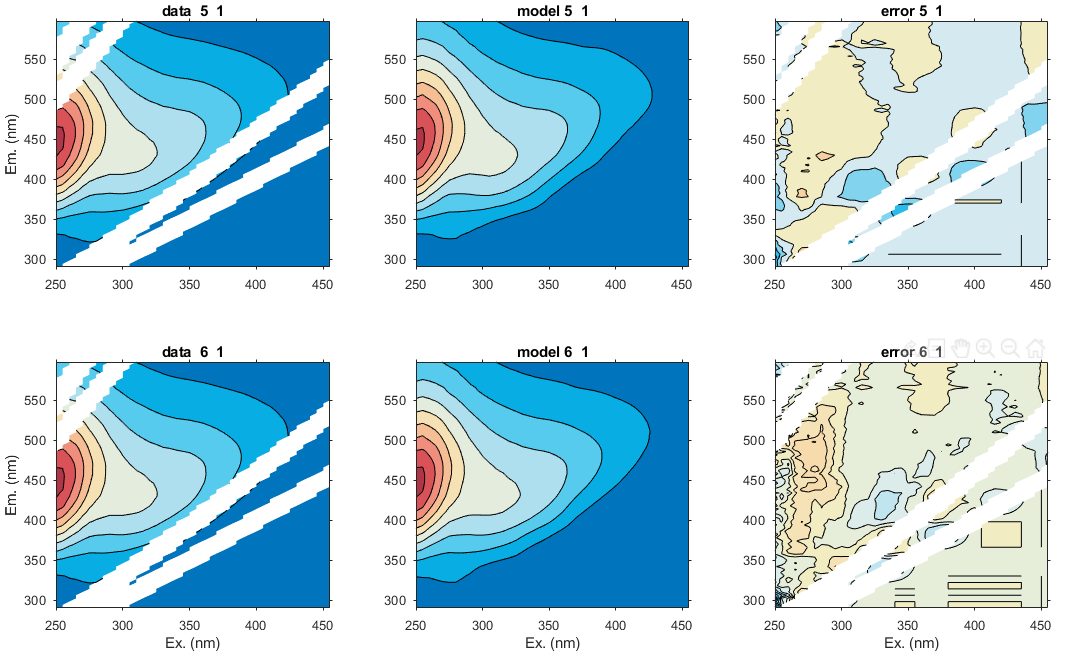It is also possible to compare the residuals for 3 or more models at the same time. See drEEM_tutorial or eemview for examples.

We can tentatively evaluate the feasibility of the current PARAFAC models. Compare the spectra for the 5- to 7-component models. Do the components all look like fluorophores or are some components being used to model noise?

``````spectralloadings(Test2,5:7)
``````

A useful indication of the number of components that should be in the model can be obtained by `specsse`, which shows the effect on model fit of adding more components, expressed as the sum of squared error (SSE) for each model plotted as a function of wavelength.

``````specsse(Test2,5:7)
``````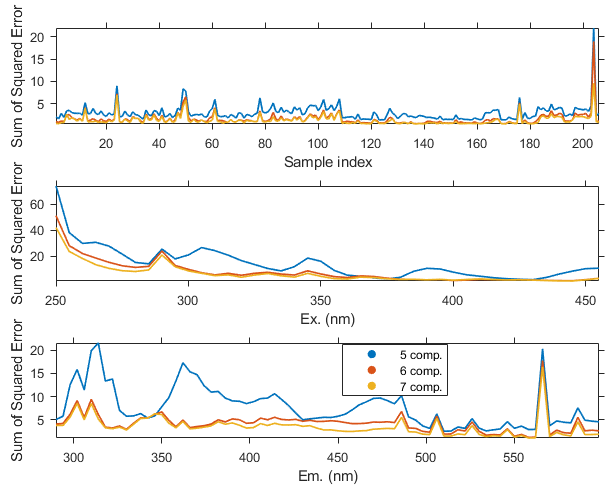Observe that the 6-component model is noticeably better (lower SSE) than the 5-component model. However, there is little difference in fit between the 6- and 7-component models. It is too soon to conclusively determine the number of components in the model, because as discussed in the next section, the solution found by N-way’s PARAFAC algorithm on any single run is not necessarily correct, particularly if the dataset is difficult to model and the convergence criterion is too lax. However, current indications for the demonstration dataset are that the solution has six components.

If you have arrived at this point in the tutorial using your own data, but have not yet obtained a fair indication of the likely number of components in the model, here are two possible paths forward:

1. Adjust and reiterate the preprocessing and exploratory modelling stages. Examine the model residuals and components for indications that contaminant peaks or residual scatter are affecting the models (e.g. very sharp peaks). Consider either excluding or re-including samples and/or wavelengths. Observe the order of preprocessing steps and start again from the top.
2. The components found by PARAFAC can vary between model runs and according to the criteria used to determine model convergence. Rerun the outlier test to see if the solutions change. If they do, the model is unstable and PARAFAC may settle on various local solutions instead of the global solution. See the tools in the next section for arriving at global solutions.

## 5. Refinement and Validation

Start by developing some new overall models. It is important to realise that PARAFAC analysis of a single dataset may produce variable results due to some procedures within the N-way PARAFAC algorithm involving random numbers. The N-way PARAFAC algorithm can therefore also converge on a local minimum in the data, instead of the true global minimum-error solution. This is especially the case when EEMs have a lot of missing data and/or when constraints are applied, regardless of how the model is initialised. When modelling does not produce a stable solution, it is an indicator that the model may have too many components and/or that more stringent (smaller) convergence criteria are needed.

To increase the chances of locating a robust solution that does not depend on the numbers used to initialise the models, a series models will be developed with each one initialised using a different random starting vector. The best (minimum error) solution will be retained as the overall model. Expect this process to take much longer than outliertest, depending on the convergence criterion used and the number of iterations requested.

To develop and compare a series of ten constrained 6-component models of a dataset with the default convergence criterion of 10e-6:

``````[LSmodel,all,details]=randinitanal(Xpre,5:7,10,'nonnegativity');
``````

Or if applying a stricter convergence criterion, e.g. 10e-8:

``````[LSmodel,all,details]=randinitanal(Xpre,5:7,10,'nonnegativity',1e-8);
``````

`LSmodel` contains only the 5-, 6-, and 7-component model with the smallest error (out of the 10 models initialized. `all` is a structure that contains all models started, and `details` contains useful information you can review that e.g. lets you know after how many iterations all of the model fits converged.

Choosing to make fewer runs will speed things up. Lowering the convergence criterion will slow it down, but may help PARAFAC to reach reasonable solutions. If `randinitanal` does not consistently produce very similar solutions (similar sum of squared residuals for each run), the model will be especially difficult to validate. See whether the model is made more stable by decreasing the convergence criterion.

`randinitanal` produces a plot showing the scores and loadings for every f-component model once all of the models have either converged (or the unfinished models have been aborted).

You can view the % of explained variance and the core consistencies with `coreandvar`.

To visualise the spectra from the least squares model:

``````spectralloadings(LSmodel,6)
comparespectra(LSmodel,6)
fingerprint(LSmodel,6)
``````

Compare the spectra for the components in the least squares model with those in the outlier test. Are they very different? If so, you may need to revisit any assumptions you have made on the basis of plotting the earlier outlier tests. Check the leverages of the least squares model for any last minute surprises and make sure you are satisfied with the residual error plots.

``````loadingsandleverages(LSmodel,6)
eemview({LSmodel, LSmodel},{'X','Model6','error_residuals'},[2 3])
``````

If you are working with a dataset that was normalised, before you export the model you will want to reverse the normalisation to obtain the unscaled scores.

``````LSmodel_r=normeem(LSmodel,'reverse',6)
``````

`LSmodel` and `LSmodel_r` are identical except that the latter contains the true (unscaled) model scores.

## 6. Split half analysis

Split half analysis is a very powerful technique for validating a PARAFAC model. However, it is computationally intensive and can take a very long time. The dataset is split into several fractions to see if the same model is obtained when modelling different groups of samples. Before attempting this, examine the loadings of the least squares model and consider whether the spectra are reasonable or not. If the spectra do not look reasonable, see if they can be improved by changing the convergence criterion and/or increasing the number of iterations. Also examine plots of the residuals for evidence of outliers or over-fitting. Often, problems that are invisible in the raw data can be very clearly seen in model residuals.

The drEEM toolbox contains a flexible routine called `splitds` that enables datasets to be divided in a range of different ways. Samples can be split in random groups, in contiguous blocks, or by assigning alternate samples to different groups. Samples can also be split according to metadata categories in order to put e.g. different sites, cruises, etc. into different splits. Also, smaller splits can be combined using `splitds` in order to create larger splits having desirable characteristics. `splitds` may seem a bit complicated at first. For help and examples, type:

``````doc splitds
``````

The function `splitds` can be used to implement the validation method suggested by Stedmon and Bro (2008, Limnol Oceanogr Meth 6, 572-579). This involves an alternating split style, in which starting from the first sample in the dataset each sample is assigned alternately to one of four splits, followed by a combination procedure where the four splits each containing a quarter of the dataset are combined in four different ways to produce four new ‘halves’ of the dataset. We will refer to this style of validation as ‘S4C4T2’ (Splits -4, Combinations -4, Tests -2). Here, we extend this method in order to assemble six different dataset ‘halves’ and produce three validation tests ‘S4C6T3’.

``````S1=splitds(Xpre,[],4,'alternating',{[1 2],[3 4],[1 3],[2 4],[1 4],[2 3]});
``````

`S1` now includes the original dataset, the six new datasets in S1.Split, and several new variables that document the splitting procedures used, i.e.:

``````			     Split: [1×6 struct]
Split_NumBeforeCombine: 4
Split_Style: 'alternating then combine'
Split_NumAfterCombine: 6
Split_Combinations: {'1  2'  '3  4'  '1  3'  '2  4'  '1  4'  '2  3'}
Split_nSample: [104 102 103 103 103 103]
``````

Now use `splitanalysis` to generate separate 5- and 6-component PARAFAC models in each dataset split. This step will take quite a while (6 x 10 x 2 models are being fit).

``````A1=splitanalysis(S1,5:6,10,'nonnegativity');
% Or (since v0.6.0)
A1=splitanalysis(S1,5:6,'starts',10,'constraints','nonnegativity');
``````

The next step is to perform a preliminary validation to see which split models are the same. In three tests we will compare the 1st and 2nd split-combinations (AB vs. CD), the 3rd and 4th (AC vs. BD) and the 5th and 6th combinations (AD vs. BC). Notice that by selecting these particular combinations, we ensure that in each test the dataset halves being compared have no samples in common.

``````splitvalidation(A1,5,[1 2;3 4;5 6],{'AB','CD','AC','BD','AD','BC'});
``````

If a model validates with `splitvalidation` it means that all of the components in the split models being compared in each test have found a match with Tucker correlation coefficient > 0.95. In this case, a message will appear requesting you complete the validation by specifying the overall least squares model. Please ignore the message for now, as this step will be done below.

Before we move on, note that it is also possible to use splitvalidation to compare all possible combinations of splits:

``````splitvalidation(A1,6);
``````

The above line of code invokes the default option where every split is compared with each of the others. But be careful and don’t lose sight of which samples went in to each split! You can NOT validate a model by comparing dataset halves that have samples in common (e.g. split combinations `[AC]` vs. `[CD]` or `[BD]` vs. `[BC]`).

If you have finished performing the split validation, you will probably have found that one or both models did not validate. In fact, if you try running `splitanalysis` several times, you may find that the solution is very unstable (it changes each time you run the analysis). When models do not validate but come close to doing so (e.g. some of the tests succeed but not others), `splitanalysis` can be run with the option to develop multiple models (keeping only the best one) and/or apply a different convergence criterion (the default is 10-6). Note that depending on how many splits there are in the dataset, on the number of runs and the convergence criterion selected, this could take a long time. To cut down on processing time, specify more runs and/or lower convergence criteria only when modelling ‘difficult’ splits (those that are least stable or most unlike the others). In each case, only the solution with the lowest error will appear in the output.

For example, run the 5-component PARAFAC analysis five times, using a convergence criterion of 10e-8:

``````A2a=splitanalysis(S1,5,'constraints','nonnegativity','starts',5,'convgcrit',1e-8)
``````

The 6-component PARAFAC solution is more stable. Run the analysis more times on difficult splits.

``````A2b=splitanalysis(S1,6,'constraints','nonnegativity','starts',5,'convgcrit',1e-8)
``````

Try the validation again:

``````splitvalidation(A2a,5);
splitvalidation(A2b,6);
``````

If this worked, you are ready to perform the 5- and 6-component model validations a final time, using the overall (least squares) model as the final input variable in splitvalidation. This time, the function produces validation plots and retrieves the spectral correlation coefficients for each split against the overall model.

Note that the validation dataset obtains scores from the overall model. Therefore, if LSmodel6 was derived from a normalised dataset (e.g. Xpre), use the reversed model (i.e. LSmodel6r) when performing the validation. This will ensure that val6 contains the true model scores.

``````val5=splitvalidation(A2a,5,[1 2;3 4;5 6],{'AB','CD','AC','BD','AD','BC'},LSmodel_r);
``````

The contents of each validated model include fields tracking the results of the validation tests and the procedures used to obtain the validation. For example, val6 contains something similar to this:

``````		Val_ModelName: 'Model6'
Val_Preprocess: 'Reversed normalisation to recover true scores'
Val_Source: 'Model6it_5'
Val_Err: 322.7535
Val_It: 692
Val_Core: 2.2518
Val_ConvgCrit: 1.0000e-06
Val_Constraints: 'nonnegativity'
Val_Initialise: 'random'
Val_PercentExpl: 99.9713
Val_CompSize: [69.9076 28.4569 29.3479 11.8407 10.0141 3.8042]
Val_Result: 'Overall Result= Validated for all comparisons'
Val_Comparisons: {'AB vs CD,' 'AC vs BD,' 'AD vs BC,'}
Val_Comparisons_Num: [3x2 double]
Val_Matches: {4x1 cell}
Val_ExCC: {4x1 cell}
Val_EmCC: {4x1 cell}
Val_Splits: {'AB' 'CD' 'AC' 'BD' 'AD' 'BC'}
Val_SplitsNum: [1 2 3 4 5 6]
``````

## 7. Export a model to Excel

PARAFAC components are often reported in terms of their peak positions. These can be calculated using `describecomp`. For example:

``````describecomp(LSmodel6,6)
``````

To convert raw PARAFAC model scores to Fmax, representing the maximum intensity of each component in each sample in the same scale as the original EEMs, use `scores2fmax`.

``````[F6,scores6]=scores2fmax(val6,6)
``````

Model results can be exported to Microsoft Excel using `modelout`. This will by default export the spectra of the chosen model, along with all the tracking information (information on data preprocessing, modelling criteria, and validation results) that are contained in the model structure being exported. It does not export the raw PARAFAC model scores, but calculates and exports Fmax (the maximum intensities of the components in the original measurement scale).

For a basic export of the val6 model:

``````modelout(val6,6,'Tutorial_Models.xls');
``````

There are two additional options for exporting models:

1. Project a larger dataset on the model to obtain Fmax for all the samples in the larger dataset.

This can be used to obtain scores for samples which were excluded (outliers) when building the model.

``````[F,B,C,Ff,P6]=modelout(val6,6, 'Tutorial_Models.xls',Xs);
``````

If projecting a dataset, beware of some caveats. First, it is essential that the new dataset differs from the modelled dataset only in the number of samples it contains. If it has different Ex or Em wavelengths, the projection will fail. Also, if it differs in the way it was preprocessed for scatter, the projection may fail or produce inaccurate results. Check in the Excel spreadsheet that Fmax for samples that were included in the model is consistent between the sheets titled `Model6Loading` and `Model6FmaxProjected`. Second, even if the modelling is successful, Fmax may be inaccurate for outlier samples because the model is inappropriate. This is possibly the reason why they had to be excluded from modelling in the first place. Extra care must be taken when interpreting the scores for outlier samples.

Look at the fit for the projected dataset. It is very poor for many of the outliers!

``````eemview({P6,P6},{'X','Model5_P','error_residuals'},[3 3],[],[],[],[1 1 0.05]);
``````

2. Export metadata for the samples included in building the model.

For example, to export the metadata for `val6`:

``````metadata4export={'cruise','site','rep','longID','ID','date','i'}
Finally, note that when the `modelout` function fails to locate some tracking information, a warning is produced. Such warnings are for informational purposes, and do not necessarily indicate there was a problem with either the analysis or export. Many of the drEEM functions produce some tracking information that is carried forward to the validated model structure, so if any of those functions were omitted when creating a particular model (or if the model being exported was not validated using drEEM), their tracking information will be absent.